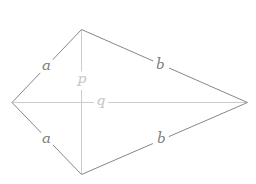# Perimeter of a Kite Formula

A kite has two pairs of equal size. Total distance around the outside is called the perimeter of a kite. This distance can be found by calculating the sum of the lengths of each pair.The perimeter formula of a kite is,

$\large Perimeter = 2a + 2b$

Where,
a = The length of the first pair
b = The length of the second pair

### Solved Example

Question: Find the perimeter of the kite whose equal sides are 18 cm and 26 cm.

Solution:

Given,
a = 18 cm
b = 26 cm

Perimeter of the kite = 2(a + b)
Perimeter of the kite = 2(18 + 26) = 2 × 44
Perimeter of the kite = 88 cm